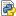Welcome to the Info TEST server!
VLACalibration.py

# VLACalibration.pyVLACalibration.py — Python Source, 6 KB (6577 bytes)

## File contents

```import os

visfile = os.getenv('MS_FILE')
workDir = os.getenv('WORK_DIR')
imageDir = os.getenv('IMAGE_DIR')
infoDir = os.getenv('INFO_DIR')
imageID = os.getenv('IMAGE_ID')

# to name the data reduction products with the same name of the ms file
basename = visfile.partition('.')

# If the name of the ms file starts with the source name, this is a convenient way to extract
sourcename = basename.partition('_')

# in this example, image products and data reduction information will be stored in subdirectories
infoName = infoDir + '/' + basename
basename = workDir + '/' + basename
splitfile = basename + '.split'
SourceImage = imageDir + '/' + sourcename + '_' + imageID

# Calibration parameters
REFANT = 'ea21'
SPW = '0:5~58'
GainTables = []

# Data processing begins
listobs(vis = visfile,
listfile = infoName + '.list')

plotants(vis = visfile,
figfile = infoName + '_antenna_layout.png')

clearstat()

flagdata(vis = visfile,
flagbackup = True,
mode = 'manual',
scan = '1')

flagdata(vis = visfile,
flagbackup = True,
mode = 'manual',
antenna = 'ea13,ea15')

flagdata(vis = visfile,
mode = 'quack',
quackinterval = 10.0,
quackmode = 'beg')

clearstat()

plotms(vis = visfile,
selectdata = True,
correlation = 'RR,LL',
averagedata = True,
avgchannel = '64',
coloraxis = 'field',
showgui = False,
plotfile = infoName + '_plotms_allscans.png')

plotms(vis = visfile,
field = '',
correlation = 'RR,LL',
timerange = '',
antenna = 'ea01',
spw = '0:31',
xaxis = 'time',
yaxis = 'antenna2',
plotrange = [-1,-1,0,26],
coloraxis = 'field',
showgui = False,
plotfile = infoName + '_plotms_datastream.png')

caltable = basename + '.antpos'

gencal(vis = visfile,
caltable = caltable,
caltype = 'antpos')

GainTables.append(caltable)

setjy(vis = visfile,
listmodels = True)

setjy(vis = visfile,
field = 'J1331+3030',
standard = 'Perley-Butler 2013',
model = '3C286_C.im',
usescratch = False,
scalebychan = True,
spw = '')

caltable = basename + '.G0'

gaincal(vis = visfile,
caltable = caltable,
field = 'J1331+3030',
refant = REFANT,
spw = '0:27~36',
calmode = 'p',
solint = 'int',
minsnr = 5,
gaintable = GainTables)

GainTables.append(caltable)

caltable = basename + '.K0'

gaincal(vis = visfile,
caltable = caltable,
field = 'J1331+3030',
refant = REFANT,
spw = SPW,
gaintype = 'K',
solint = 'inf',
combine = 'scan',
minsnr = 5,
gaintable = GainTables)

GainTables.append(caltable)

caltable = basename + '.B0'

bandpass(vis = visfile,
caltable = caltable,
field = 'J1331+3030',
spw = '',
refant = REFANT,
combine = 'scan',
solint = 'inf',
bandtype = 'B',
gaintable = GainTables)

GainTables.append(caltable)

caltable = basename + '.G1'
G0 = basename + '.G0'
GainTables.remove(G0)

gaincal(vis = visfile,
caltable = caltable,
field = 'J1331+3030',
spw = SPW,
solint = 'inf',
refant = REFANT,
gaintype = 'G',
calmode = 'ap',
solnorm = False,
gaintable = GainTables,
interp = ['linear','linear','nearest'])

gaincal(vis = visfile,
caltable = caltable,
field = 'J1822-0938',
spw = SPW,
solint = 'inf',
refant = REFANT,
gaintype = 'G',
calmode = 'ap',
gaintable = GainTables,
append = True)

gaincal(vis = visfile,
caltable = caltable,
field = 'J0319+4130',
spw = SPW,
solint = 'inf',
refant = REFANT,
gaintype = 'G',
calmode = 'ap',
gaintable = GainTables,
append = True)

GainTables.append(caltable)

alpha = log(7.53261/7.6677)/log(4663.0/4536.0) # Spectral index computed from setjy
i0=7.6677 # Stokes I value for spw 0 ch 0
c0=0.112 # Fractional polarization=11.2%
d0=33*pi/180 # polarization angle of 33 degrees converted to radians

setjy(vis = visfile,
field = 'J1331+3030',
standard = 'manual',
spw = '0',
fluxdensity = [i0,0,0,0],
spix = [alpha,0],
reffreq = '4536.0MHz',
polindex = [c0,0],
polangle = [d0,0],
scalebychan = True,
usescratch = False)

caltable = basename + '.Kcross'

gaincal(vis = visfile,
caltable = caltable,
field = 'J1331+3030',
spw = SPW,
gaintype = 'KCROSS',
solint = 'inf',
combine = 'scan',
refant = REFANT,
gaintable = GainTables,
gainfield = ['','','','J1331+3030'],
parang = True)

GainTables.append(caltable)

caltable = basename + '.D1'

polcal(vis = visfile,
caltable = caltable,
field = 'J0319+4130',
spw = SPW,
refant = REFANT,
poltype = 'Df',
solint = 'inf',
combine = 'scan',
gaintable = GainTables,
gainfield = ['','','','J0319+4130',''])

caltable = basename + '.D2'

polcal(vis = visfile,
caltable = caltable,
field = 'J0319+4130',
spw = SPW,
refant = REFANT,
poltype = 'Df+QU',
solint = 'inf',
combine = 'scan',
gaintable = GainTables,
gainfield = ['','','','J1822-0938',''])

GainTables.append(caltable)

caltable = basename + '.X1'

polcal(vis = visfile,
caltable = caltable,
field = 'J1331+3030',
poltype = 'Xf',
solint = 'inf',
combine = 'scan',
gaintable = GainTables,
gainfield = ['','','','J1331+3030','',''])

GainTables.append(caltable)

caltable = basename + '.G1'
fluxtable = basename + '.fluxscale1'

fluxscale(vis = visfile,
caltable = caltable,
fluxtable = fluxtable,
reference = ['J1331+3030'],
transfer = ['J1822-0938,J0319+4130'],
incremental = False)

GainTables.remove(caltable)
GainTables.insert(1, fluxtable)

fields = ['J1331+3030', 'J0319+4130', 'J1822-0938']

for item in fields:
applycal(vis = visfile,
field = item,
gaintable = GainTables,
gainfield = ['',item,'','','','',''],
interp = ['','nearest','','','','',''],
calwt = [False],
parang = True)

applycal(vis = visfile,
field = '2~8',
gaintable = GainTables,
gainfield = ['','J1822-0938','','','','',''],
interp = ['','linear','','','','',''],
calwt = [False],
parang = True)

split(vis = visfile,
outputvis = splitfile,
datacolumn = 'corrected',
field = '2~8')

statwt(vis = splitfile)

clean(vis = splitfile,
imagename = SourceImage,
field = '',
spw = '',
mode = 'mfs',
niter = 20000,
gain = 0.1,
threshold = '3.0mJy',
psfmode = 'clark',
imagermode = 'mosaic',
ftmachine = 'mosaic',
multiscale = ,
interactive = False,
mask = 'circle [ [240pix, 240pix], 120pix]',
imsize = [480,480],
cell = ['2.5arcsec','2.5arcsec'],
stokes = 'I',
weighting = 'briggs',
robust = 0.5,
pbcor = False,
usescratch = True)
```